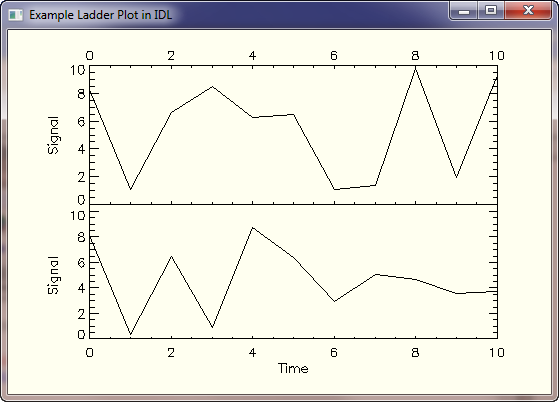QUESTION: I'm trying to make a stacked or ladder plot in IDL, in which one plot is stacked on top of the other. I've been trying to use !P.Multi but I'm not having any luck. Is there a way to do this in IDL?ANSWER: Yes, but forget !P.Multi. What you want to do is position the plot axes with the Position keyword and add subsequent plots with the NoErase keyword. And to keep the space between plots right, so you don't have to be overly concerned with the axis annotation, it is good to add a tiny bit of space between plots that can be filled in with the PLOTS command. Here is some code that will produce a very simple, two plot ladder plot. Of course, you can add as many plots as you like by following this example.

```   Plot, RandomU(seed, 11) * 10, XStyle=8, Position=[0.15, 0.15, 0.9, 0.50], \$
XTitle='Time', YTitle='Signal'
Plot, RandomU(seed, 11) * 10, Position=[0.15, 0.52, 0.9, 0.90], \$
/NoErase, XTickformat='(A1)', YTitle='Signal'
Plots, [0.15, 0.15], [0.50, 0.52], /Normal ; Fix left axis.
Plots, [0.90, 0.90], [0.50, 0.52], /Normal ; Fix right axis.
```

You see an example in the figure below.Sometimes you wish the top axis to look like the bottom axis. If that is the case, you have to use the AXIS command to repair the top of the last plot. The code will look like this.

```   Plot, RandomU(seed, 11) * 10, XStyle=8, Position=[0.15, 0.15, 0.9, 0.50], \$
XTitle='Time', YTitle='Signal'
Plot, RandomU(seed, 11) * 10, Position=[0.15, 0.52, 0.9, 0.90], \$
/NoErase, XTickformat='(A1)', YTitle='Signal', XStyle=8
Plots, [0.15, 0.15], [0.50, 0.52], /Normal ; Fix left axis.
Plots, [0.90, 0.90], [0.50, 0.52], /Normal ; Fix right axis.
Axis, !X.CRange, !Y.CRange, XRANGE=!X.CRANGE, XAXIS=1
```

You see an example in the figure below.JD Smith has written a routine named GANG_PLOT_POS to help you get the positions for an arbitrary number of stacked plots. It doesn't work exactly in the manner as the one described above, but is a similar approach and worth trying out.